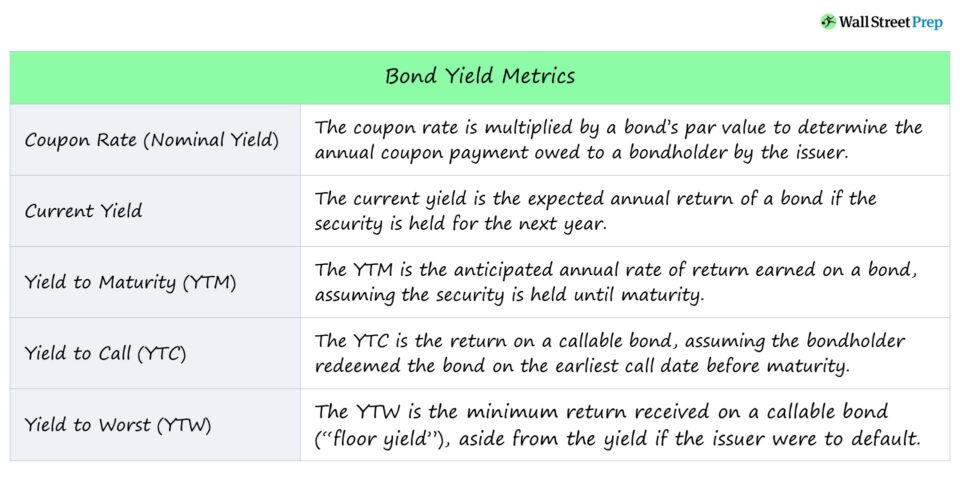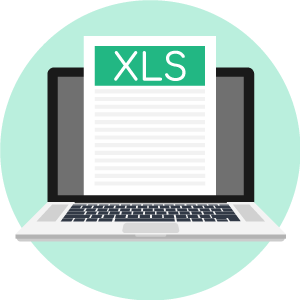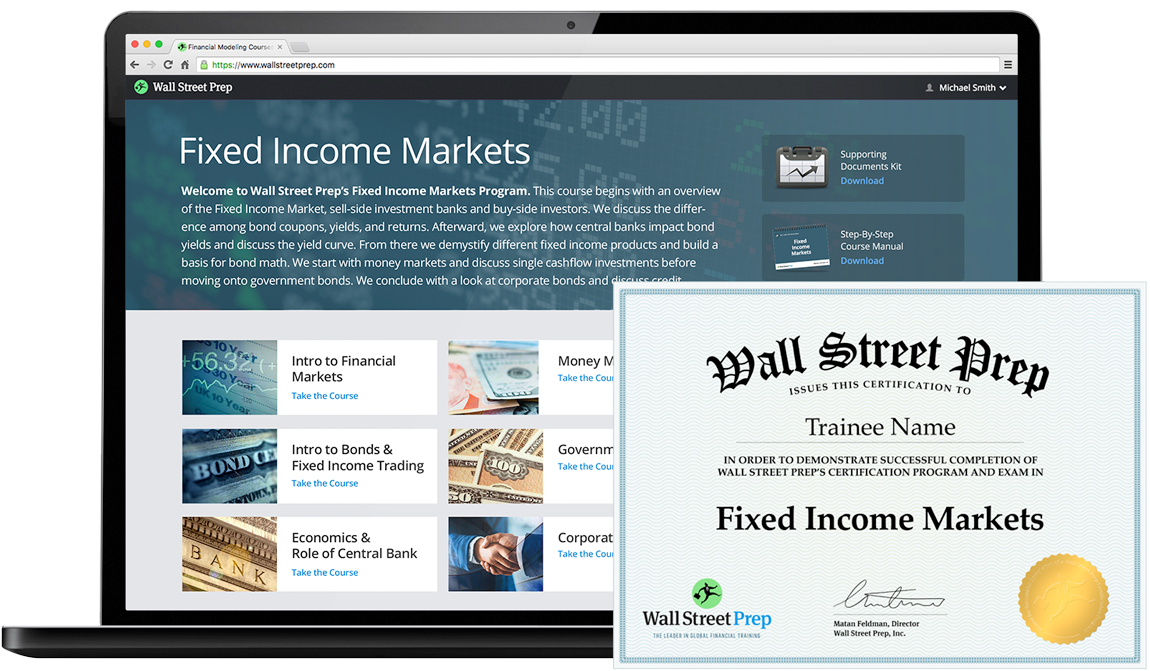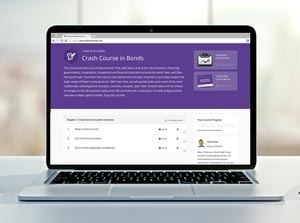# Bond Yield

Guide to Understanding Bond Yields• What are the formulas for calculating the common bond yield metrics?
• How does the current yield, yield to maturity (YTM), yield to call (YTC), and yield to worst (YTW) differ in the return measured?
• How are bond prices, yields, and interest rates related?
• What happens to a bond’s yield when bond prices rise?

## Bond Yield Types

The bond yield earned by bondholders can be measured using several different approaches, each coming with its own set of pros/cons.

By not relying on only a single method to arrive at the yield on a bond, bondholders can see a complete picture of the bond’s risk/return profile.

Below are the common bond yield calculations that we’ll discuss:

## Bond Yield Determinants

The factors that play an integral role in determining the yield on bonds are the following:

• Face Value: The par value of the bond on the date of issuance, which the coupon is based on.
• Coupon Rate: The interest rate pricing on the bond determines the dollar amount of coupon payments due each year.
• Coupon: The annual interest amount owed to the bondholder by the issuer.
• Maturity: The tenor (i.e. length of time) of the bond issuance, as agreed upon by the issuer and the investor.

## Bond Price vs Bond Yield Relationship

Bond prices and bond yields are inversely related – so if the price of a bond goes up, its yield declines (and vice versa).

Notably, the factor with arguably the most influence on bond yields is the prevailing interest rate environment.

The general rule of thumb is that interest rates and yield have an inverse relationship, i.e. if interest rates rise, bond prices decline (and vice versa).

• If interest rates rise, the prices of bonds in the market fall, causing bond yields to increase (i.e. higher coupon).
• If interest rates decline, the prices of bonds in the market rise, resulting in bond yields falling (i.e. lower coupon)

Coupon Rate of Bond

The coupon rate, also known as the “nominal yield,” determines the annual coupon payment owed to a bondholder by the issuer until maturity.

The coupon, i.e. the annual interest payment, equals the coupon rate multiplied by the bond’s par value.

### Bond Coupon Rate Formula

The coupon rate can be calculated by dividing the annual coupon payment by the bond’s par value.

• Coupon Rate = Coupon / Bond Par Value

For example, given a \$1,000 par value and bondholder entitled to receive \$50 per year, the coupon rate is 5%.

• Coupon Rate = \$50 / \$1,000
• Coupon Rate = 5%

Whereas yields move along with the market, coupons are distinct in that they remain fixed during the bond’s term.

Regardless of the changes in the market price of a bond, the coupon remains constant, unlike the other bond yields, which we’ll discuss in more detail in the subsequent sections.

## Current Yield of Bond

The coupon rate (“nominal yield”) represents a bond’s annual coupon divided by its face (par) value and is the expected annual rate of return of a bond, assuming the investment is held for the next year.

### Current Yield Formula

To calculate the current yield, the formula consists of dividing the annual coupon payment by the current market price.

• Current Yield = Annual Coupon / Bond Price

Calculating the current yield of a bond is a three-step process:

• Step 1: The current bond price can be readily observed in the markets – in which the bond can either trade at a discount, at par or at a premium to par.
• Step 2: The annual coupon is a function of the bond’s coupon rate, par value, and payment frequency – and, if applicable, the coupon rate must be annualized.
• Step 3: The current yield formula equals the annual coupon payment divided by the bond’s current market price, expressed as a percentage.

For example, a bond trading at \$900 with a \$1,000 face value and a \$60 coupon has a 6% coupon rate and a current yield of 6.7%.

Unlike the coupon rate, which remains fixed, the current yield fluctuates based on the market price of the bonds.

Note that the current yield metric only becomes relevant if the market price of the bond deviates from its par value.

## Yield to Maturity (YTM) of Bond

The yield to maturity (YTM) is the rate of return received if the investment is held to maturity, with all interest payments reinvested at the same rate as the YTM.

If bond investors use the term “yield,” in all likelihood, they are most likely referring to the yield to maturity (YTM).

YTM accounts for all interest received over time, the initial purchase price (i.e. capital invested), the par value (i.e. amount returned at redemption), and maturity (i.e. the time from issuance to maturity).

The widespread usage of YTM is largely attributable to how the metric can be used for comparisons among bonds with different maturities and coupons.

Unlike the yield to maturity (YTM), the current yield is not the “real” yield of a bond since it neglects the yield associated with recovering the principal amount and assuming the reinvestment of coupon payments at the same rate as the YTM.

## Discount, Par & Premium Bond

Since the price of a bond adjusts based on the prevailing macro conditions and credit health of the underlying issuer (e.g. credit ratings), bonds can be purchased at discounts or premiums relative to par.

Moreover, the current market prices of bonds can be categorized into three buckets:

1. Discount Bond: YTM > Coupon Rate
2. Par Bond: YTM = Coupon Rate
3. Premium Bond: YTM < Coupon Rate

For example, if the par value of a bond is \$1,000 (“100”) and if the price of the bond is currently \$900 (“90”), the security is trading at a discount, i.e. trading below its face value.

Conversely, if the bond price in the market is \$1,100 (“110”), the bond is selling at a premium, i.e. priced by the market above its face value.

###### Bond Pricing Impact on Current Yield / Coupon Rate

The pricing of the bond (e.g. discount, par, premium) has a direct impact on the current yield and coupon rate, as described below:

• Bond Price < Par Value → “Discount” Bond
• Discount Bond: Current Yield > Coupon Rate
• Bond Price = Par Value → “Par” Bond
• Par Bond: Current Yield = Coupon Rate
• Bond Price > Par Value → “Premium” Bond
• Premium Bond: Current Yield < Coupon Rate

## Callable Bonds Features

Callable bonds should exhibit greater yields than comparable, non-callable bonds – all else being equal.

Before delving into yield to call (YTC) and yield to worst (YTW), it would be best to preface the sections with a review of callable bonds.

If a bond issuance is callable, the issuer can redeem the borrowing before maturity, i.e. pay off the debt earlier.

Within the bond indenture of callable bonds, the contract will state the schedule of when prepayment is permitted. For example, the “NC/3” abbreviation means the bond issuer cannot redeem the bonds until three years have passed.

Certain provisions included in the bond agreement can make yield calculations more complicated, which is the call feature in this scenario.

• Call Price: The set fixed cost to redeem the bonds, typically set at a slight premium above the par value.
• Call Premium: Common bond feature makes the offering more attractive to risk-averse investors, i.e. downside risk protection.
• Prepayment Fees: The call provision can also result in prepayment fees, which again, are intended to make the bond offering more marketable when raising capital from prospective investors.

Specific to callable bonds, one concern during declining interest rates is that the bond’s issuer might view the low interest rates as a chance to refinance its existing debt at more favorable rates.

## Yield to Call (YTC)

The yield to call (YTC) metric implies that a callable bond was redeemed (i.e. paid off) sooner than the stated maturity date.

Yield to call (YTC) is the anticipated return on a callable bond, assuming that the bondholder redeemed (i.e. retired) the bond on the earliest call date.

The YTC metric is only applicable to callable bonds, in which the issuer has the right to redeem the bonds earlier than the stated maturity date.

Issuers are more likely to call a bond earlier than scheduled due to:

• Refinancing Debt in a Lower Interest Rate Environment
• Reducing the Proportion of Debt in the Capital Structure

Side Note: While it is possible to calculate the YTC on a date later than the first call date, it is standard to calculate the metric using the first date on which the bond can be redeemed.

###### Yield to Maturity (YTM) vs Yield to Call (YTC)

The distinctions between YTM and YTC are as follows:

• YTC uses the call date rather than the date of maturity.
• YTC calculates the return if the bonds are redeemed, i.e. prior to maturity.
• YTC uses the call price rather than the par value as the redemption price.

## Yield to Worst (YTW)

Yield-to-worst (YTW) is the lowest potential return received by a lender (i.e. the most conservative yield), as long as the issuer does not default.

Assuming the issuer does not default, the yield to worst (YTW) is the minimum return received on a callable bond – assuming the issuer does not default.

In other words, YTW is the lowest potential yield – i.e. “floor yield” – aside from the expected yield if the issuer were to default on the debt obligation.

As part of worst-case scenario contingency planning, the yield to worst (YTW) is estimated – but note that “worst-case” here refers to the bond being redeemed by the issuer at the earliest possible date, rather than a default.

From determining the yield to worst (YTW), bondholders can mitigate their downside risk by avoiding being unexpectedly blindsided by an issuer calling a bond early.

In real-life, the yield to worst (YTW) is applicable only for callable bonds and those trading at a premium.

## Bond Yield Calculator – Excel Template

We’ll now move to a modeling exercise, which you can access by filling out the form below.Submitting ...

## Bond Yield Example Calculation Assumptions

In our illustrative exercise, we’ll calculate the yield on a bond using each of the metrics discussed earlier.

Suppose the bond issuance has a maturity of five years, and the financing was finalized on 12/31/2021 with the first call date two years after the settlement date.

• Settlement Date: 12/31/21
• Maturity Date: 12/31/26
• First Call Date: 12/31/23 (“NC/2”)

There will be three distinct scenarios in which all the assumptions will be identical except for the current market pricing.

Given a par value of \$1,000 (“100”), the prices for each scenario are as follows:

1. Discount Bond: \$900 (“90”)
2. Par Bond: \$1,000 (“100”)

We’ll assume the bond pays an annual coupon at an interest rate of 8.5%, so the annual coupon is \$60.

• Frequency of Coupon: 2
• Coupon Rate: 8.5%
• Annual Coupon: \$85

## Bond Current Yield Calculation

For our first returns metric, we’ll calculate the current yield by multiplying the coupon rate (%) by the par value of the bond (“100”), which is then divided by the current bond quote.

Current Yield = (Coupon Rate * Par Value) / Bond Quote

• Current Yield – Discount: 9.44%
• Current Yield – Par: 8.50%
• Current Yield – Premium: 7.73%

Side Note: If the bond quote convention is followed (e.g. 100 par value), all figures should utilize the same convention, or the formula will not work properly.

###### YIELD Excel Function

Now, we will enter our assumptions into the Excel “YIELD” function to calculate the yield to maturity (YTM) and yield to call (YTC).

• YIELD Excel Function “= YIELD (settlement, maturity, rate, pr, redemption, frequency)”

Where:

• settlement = Settlement Date
• maturity = Original Maturity Date or Early Redemption Date
• rate = Annual Coupon Rate
• pr = Bond Quote (% of Par)
• redemption = Par Value or Call Price
• frequency = Number of Compounding Periods (Annual = 1, Semi-Annual = 2)

## Bond Yield to Maturity (YTM) Calculation

The inputs for the yield to maturity (YTM) formula in Excel are shown below.

Yield to Maturity (YTM): “= YIELD (12/31/2021, 12/31/2026, 8.5%, Bond Quote, 100, 2)”

• Yield to Maturity (YTM) – Discount: 11.16%
• Yield to Maturity (YTM) – Par: 8.50%
• Yield to Maturity (YTM) – Premium: 6.15%

## Bond Yield to Call (YTC) Calculation

Moving on, the yield to call (YTC) is virtually identical – but “maturity” is changed to the first call date and “redemption” to the call price, which we’ll assume is set at “104.”

Yield to Call (YTC): “= YIELD (12/31/2021, 12/31/2023, 8.5%, Bond Quote, 104, 2)”

The call price assumption of “104” is the quoted bond price that the issuer must pay to redeem the debt issuance entirely or partially, earlier than the actual maturity date.

• Yield to Call (YTC) – Discount: 16.33%
• Yield to Call (YTC) – Par: 10.35%
• Yield to Call (YTC) – Premium: 5.10%

## Bond Yield to Worst (YTW) Calculation

The final step is to calculate the yield to worst (YTW), which is the lower value between the yield to maturity (YTM) and the yield to call (YTC).

The “MIN” Excel function outputs the lower value between the two.

Yield to Worst (YTW) = MIN (Yield to Maturity, Yield to Call)

• Yield to Worst (YTW) – Discount: 11.16%
• Yield to Worst (YTW) – Par: 8.50%
• Yield to Worst (YTW) – Premium: 5.10%

Aside from the premium bond, the yield to worst (YTW) is equal to the yield to call (YTM). The issuer of a premium bond is likely to redeem the bond earlier, especially if interest rates have declined.Globally Recognized Certification Program

#### Get the Fixed Income Markets Certification (FIMC©)

Wall Street Prep's globally recognized certification program prepares trainees with the skills they need to succeed as a Fixed Income Trader on either the Buy Side or Sell Side.Inline FeedbacksLearn Online: Crash Course in Bonds

For those pursuing fixed income research, investments, sales and trading or investment banking.

X

The Wall Street Prep Quicklesson Series

7 Free Financial Modeling Lessons

Get instant access to video lessons taught by experienced investment bankers. Learn financial statement modeling, DCF, M&A, LBO, Comps and Excel shortcuts.